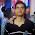### Pebble Placement Puzzle 1

Source: AUSTMS Gazette 35

Problem:

There are several pebbles placed on an n × n chessboard, such that each pebble is inside a square and no two pebbles share the same square.

Perry decides to play the following game. At each turn, he moves one of the pebbles to an empty neighboring square. After a while, Perry notices that every pebble has passed through every square of the chessboard exactly once and has come back to its original position.

Prove that there was a moment when no pebble was on its original position.

1.Assume there are M stones in n x n board (M < nxn). WLOG assume that M is the last stone which will be moved from its original place(whenever it may be moved).
Since the rest M-1 stones have not yet occupied the "Original Position" of M, neither one has returned to their respective original positions.
All the M-1 stones are not in their respective original positions.
Thus when M is moved the current positions of all the pebbles will be different from their original positions.

1.2.For the first case, If we assume that the number of empty cells are more than the number of pebbles then after the first round, none of the pebbles will be in their original position

For the second case, when the number of free cells are less than the number of pebbles, even if there is only one empty space left, that space will traverse throughout until all the pebbles have been moved. At this point none of the pebbles are in their original position

3.Let, "x" be the first element to visit all the squares exactly once and return to it's original position. The claim is that no other stone can be in it's original position in the state when x is about to enter it's original position. If y is in it's original position just before x is about to enter, then "y" never left it's original position, since "x" is the first element to return. If "y" never left, "x" could not have visited y's cell, thus a contradiction. So no stone is in it's original position